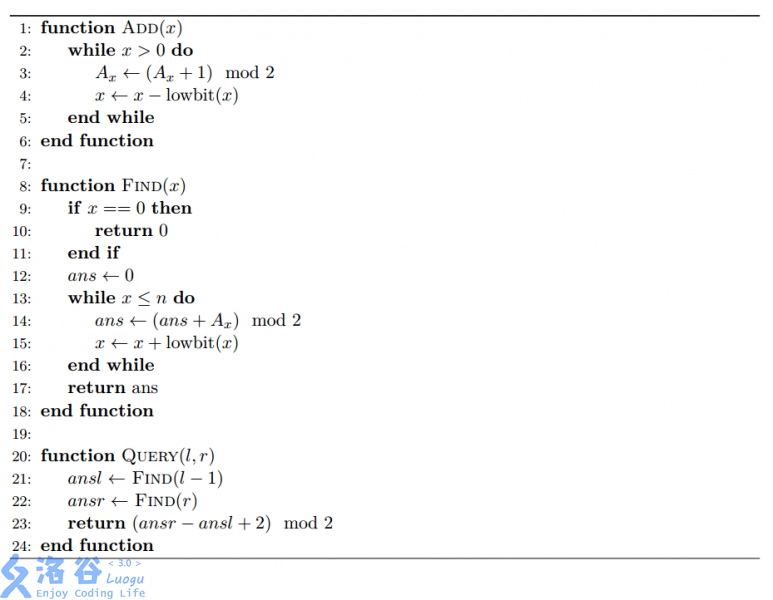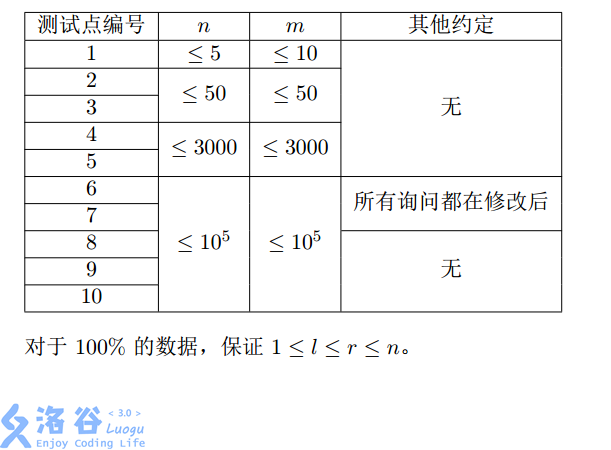P3688 [ZJOI2017]树状数组

• 217通过
• 1.2K提交
• 题目提供者 ARZhu
• 评测方式 云端评测
• 标签 二维线段树 树状数组 线段树 各省省选 2017 浙江 O2优化 高性能
• 难度 省选/NOI-
• 时空限制 4000ms / 512MB
• 提示：收藏到任务计划后，可在首页查看。

题目描述

漆黑的晚上，九条可怜躺在床上辗转反侧。难以入眠的她想起了若干年前她的一次悲惨的OI比赛经历。那是一道基础的树状数组题。

给出一个长度为$n$的数组$A$，初始值都为0，接下来进行$m$次操作，操作有两种：

* 1 x，表示将 $A_{x}$ 变成 $\left ( A_{x}+ 1 \right )$ mod 2。

* 2 l r，表示询问 $\left ( \sum_{i=l}^{r} A_{i} \right )$ mod 2。

尽管那个时候的可怜非常的 simple，但是她还是发现这题可以用树状数组做。当时非常young 的她写了如下的算法：其中 lowbit($x$) 表示数字 $x$ 最低的非 0 二进制位，例如 lowbit(5) = 1, lowbit(12) = 4。进行第一类操作的时候就调用 Add($x$)，第二类操作的时候答案就是 Query($l$,$r$)。

如果你对树状数组比较熟悉，不难发现可怜把树状数组写错了： Add 和 Find 中 $x$ 变化的方向反了。因此这个程序在最终测试时华丽的爆 0 了。

然而奇怪的是，在当时，这个程序通过了出题人给出的大样例——这也是可怜没有进行对

拍的原因。

现在，可怜想要算一下，这个程序回答对每一个询问的概率是多少，这样她就可以再次的

感受到自己是一个多么非的人了。然而时间已经过去了很多年，即使是可怜也没有办法完全回忆起当时的大样例。幸运的是，她回忆起了大部分内容，唯一遗忘的是每一次第一类操作的 $x$

的值，因此她假定这次操作的 $x$ 是在 $\left [ l_{i},r_{i} \right ]$ 范围内 等概率随机 的。

具体来说，可怜给出了一个长度为 $n$ 的数组 $A$，初始为 0，接下来进行了 $m$ 次操作：

* 1 $l$ $r$，表示在区间 $\left [ l, r \right ]$ 中等概率选取一个 $x$ 并执行 Add($x$)。

* 2 $l$ $r$，表示询问执行 Query$\left ( l, r \right )$得到的结果是正确的概率是多少。

输入输出格式

输入格式：

第一行输入两个整数 $n,m$。

接下来 $m$ 行每行描述一个操作，格式如题目中所示。

输出格式：

对于每组询问，输出一个整数表示答案。如果答案化为最简分数后形如 $\frac{x}{y}$，那么你只需要输出 $x \times y^{-1}$ mod 998244353 后的值。（即输出答案模998244353）。

输入输出样例

输入样例#1： 复制
5 5
1 3 3
2 3 5
2 4 5
1 1 3
2 2 5
输出样例#1： 复制
1
0
665496236

说明

样例说明

在进行完 Add(3) 之后， A 数组变成了 [0, 1, 1, 0, 0]。所以前两次询问可怜的程序答案都是1，因此第一次询问可怜一定正确，第二次询问可怜一定错误。

大数据详见大数据

时空限制

时间限制4s，空间限制512M

数据范围更新：2018/05/13 @larryzhong 提供了5组强的数据。

提示
标程仅供做题后或实在无思路时参考。
请自觉、自律地使用该功能并请对自己的学习负责。
如果发现恶意抄袭标程，将按照I类违反进行处理。